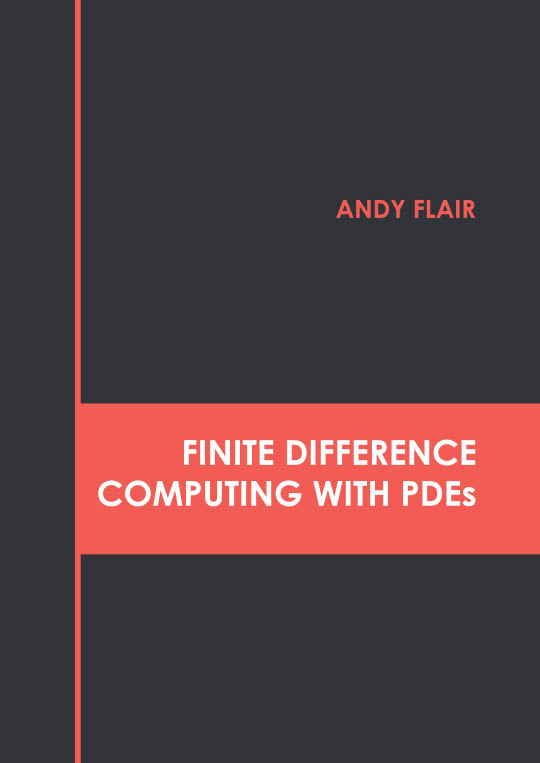# Books /Finite Difference Computing with PDEs

BROWSE BY

### Finite Difference Computing with PDEs9781639892037

Author

Andy Flair

English

##### Publication Year

(2022)

Category :

Mathematics and Statistics - Differential Equations

\$ 154.95

##### Description

An equation that relates to one or more functions with its derivatives is called a differential equation. A partial differential equation (PDE) is a type of differential equation, in which the equation consists of unknown multi variables with their partial derivatives. This is a special case of an ordinary differential equation. There is a large amount of modern mathematical and scientific research on methods for numerically approximating solutions of particular PDEs using computers. These are numerical methods for approximating the solutions to differential equations using finite difference equations to approximate derivatives. Finite difference computing is one of the most widely used methods for solving PDEs. This book outlines the processes and applications of finite difference computing with PDEs in detail. The topics included herein on finite difference computing with PDEs are of utmost significance and bound to provide incredible insights to readers. The book is appropriate for students seeking detailed information in this area as well as for experts.